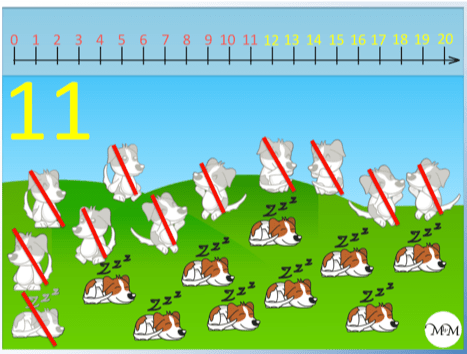# Counting to Ten using a Number Line

Counting to Ten using a Number Line• We always start at zero, which is at the beginning of our number line.
• To count the birds that have appeared, we can point at each bird and say the number as we count it.
• We cross off each bird that we count to make sure we don’t count it again.
• Once all birds have been crossed off, this is the number of birds that we have.
• We have three birds in total.
Only say one number as you count each object.

Cross it off after you count it.• Originally there are zero snakes, so we start at zero on our number line.
• Some snakes appear, which we will count.
• Remember to point at each snake that we count and say one number with each snake we point at.
• We cross off each snake after we have counted it and said its number.
• We have: 1, 2, 3, 4, 5, 6, 7, 8 snakes.
• We move one place along our number line every time we say the next number.
• We have crossed off all of the snakes after we have said the number 8.
• Since the number 8 was the last number we said, we have eight snakes.Supporting Lessons# Teaching your Child to Count to Ten using a Number Line

In this lesson we are going to learn how to count to ten. We will be looking at some examples that will help you teach your child to count.

We will be counting the numbers of animals that we have in these examples. We will look at numbers ranging from zero to ten, using a number line to help us.

We are going to count the number of birds in the following example:Before we even start counting any of the birds, we first start at zero on our number line.

When teaching your child to count, it is helpful to tell them that we first start at zero. You could ask them to put their finger on the zero place on the number line.

As we count the birds, we will move along the number line, one number at a time.

We would certainly recommend your child knowing how to say the numbers one to ten in order by memory before actually counting anything.

If your child is familiar (preferably fluent) with saying the sequence:

1, 2, 3, 4, 5, 6, 7, 8 , 9, 10

verbally, then the actual process of counting will be much easier.

If we were counting physical items, we could always move each item to one side as we count it, however we will not always be able to do this.

When teaching your child to count, we can consider some important strategies to help them.

Only say one number for each object that we count (it can be helpful for your child to physically point at each bird as they say each number).

If they say more than one number as they count one bird, start again.

We will cross out each bird after we count it and say the number, so that we don’t count the same bird more than once. We say ‘one’ as we point at the bird and cross it off afterwards.We count the next bird by moving along the number line. We count two.Next, we count the final bird by moving along the number line to three.

We cross off this bird after we say the number ‘three’.

Since we have crossed off all of the birds, we stop. ‘Three’ was the last number that we said, therefore there are three birds altogether.It would be worth showing your child the same process as we move along the number line at the top.

We counted the birds by starting at zero and moving one place along the number line for every bird. We counted one, two, three.In the next example, we will count the number of dogs.

Remember that we start at zero and it is worth saying zero with your child before you start counting.

We count the first dog as ‘1’ and cross it out after we say the number.

We count the next dog as ‘2’ and cross it out after we say the number.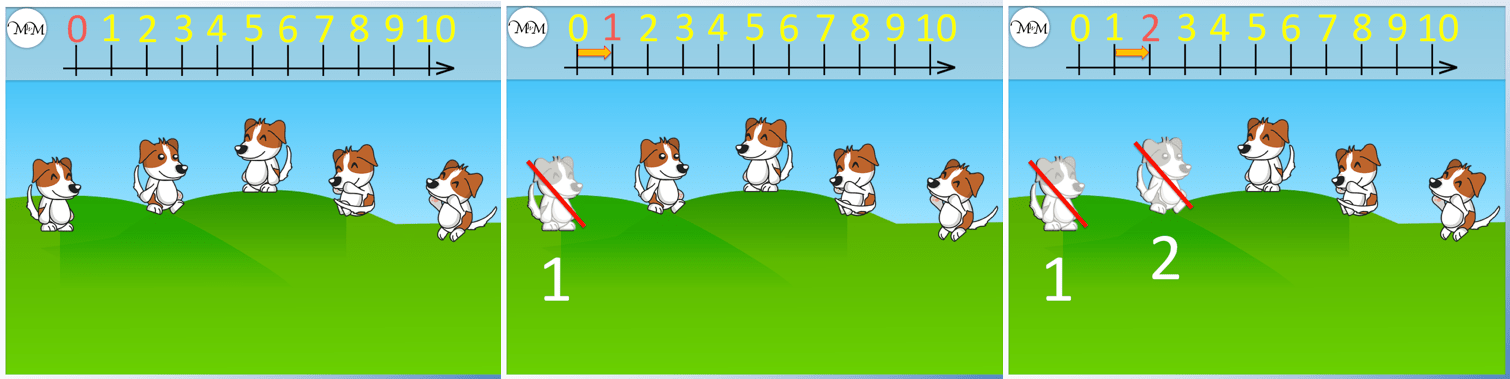We count ‘3’ and cross out the next dog after we say the number.

We count ‘4’ and cross out another dog.

We count ‘5’ and cross out the final dog.

There are five dogs altogether. Remember once we have crossed out all of the animals, we can stop counting.When teaching your child to count drawn objects, you could cross out drawings on paper. If you are teaching your child to count physical counters, you could move the counters as you count them.

As your child practises counting, we slowly move on to counting without crossing out and saying the number out loud as their eyes track to the next object, rather than pointing.

In the final counting example, we will count the number of snakes.

Again, we will start at zero and move one place along the number line for each snake that we count.

We count ‘1’ and cross out a snake.

We count ‘2’ and cross out another snake.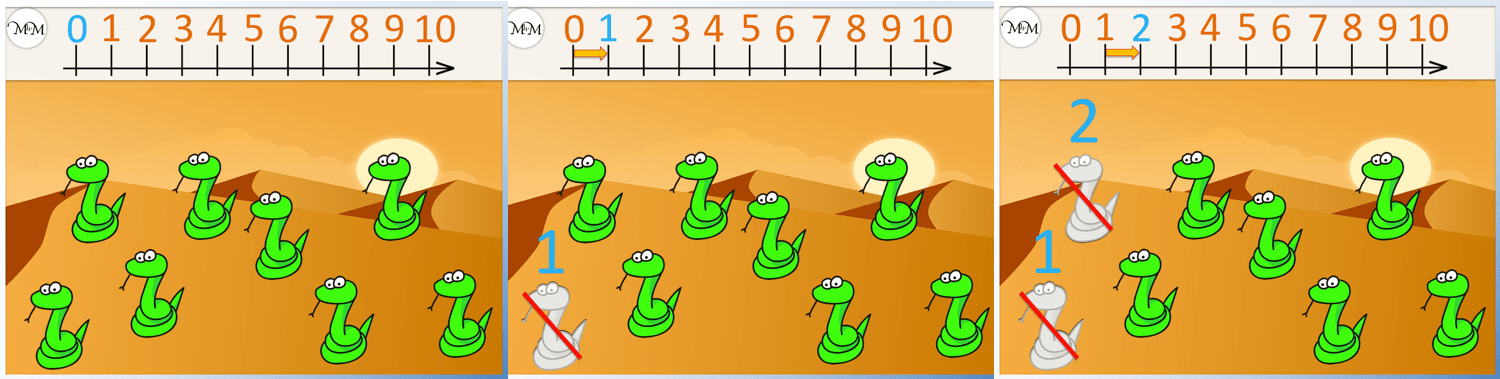We count ‘3’ and cross out a snake.

We count ‘4’.

We count ‘5’.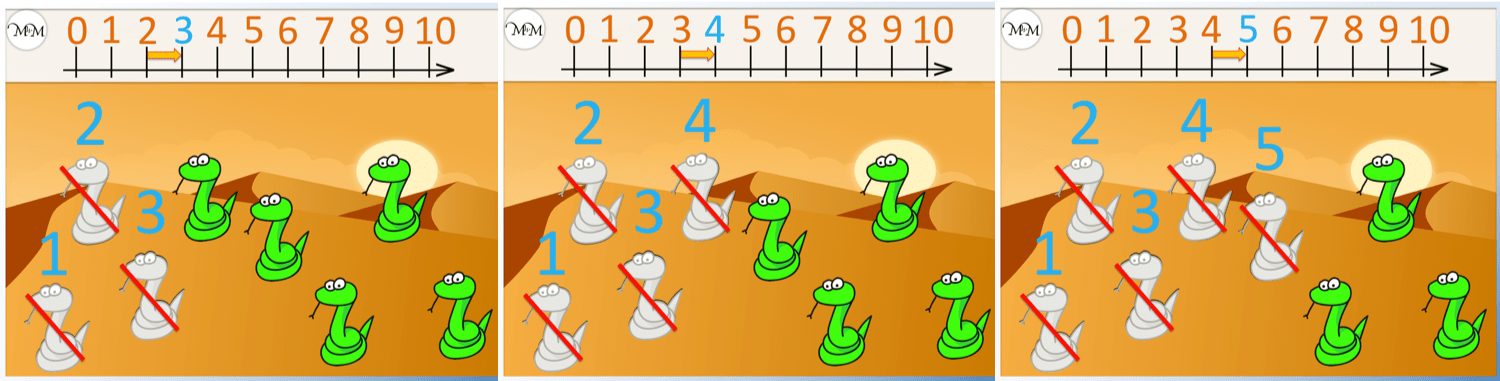We count ‘6’.

We count ‘7’.

Finally, we count ‘8’.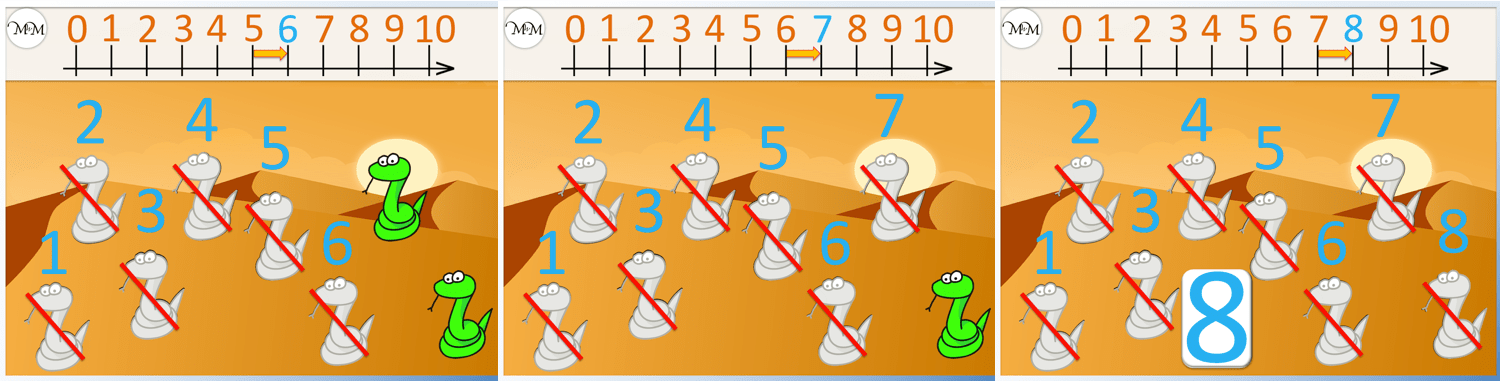We have now counted all of the snakes. Therefore, there are eight snakes altogether.Now try our lesson on Counting to 20 using a Number Line where we learn how to count on from ten, counting the numbers to twenty.